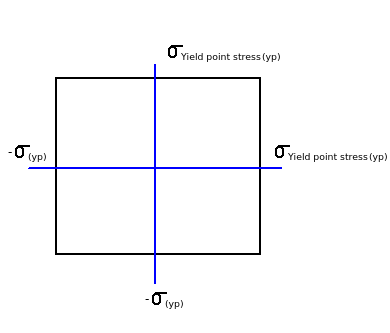# What is Rankine's theory?

Q.  In simple tensile test, when maximum principle stress reaches the value of yield point, the material subjected to complex stresses fail. This theory is called as ________.
- Published on 21 Sep 15

a. Coulumb's theory
b. Rankine's theory
c. Venant's theory
d. Von Mises theory

#### Discussion

• Sravanthi   -Posted on 25 Nov 15
- Different theories of failures are developed depending upon physical behavior of materials.

- Maximum principal stress theory is one of the theory which is also called as Rankine's theory.

- According to this theory, in simple tensile test, when maximum principle stress reaches the value of yield point, the material subjected to complex stresses fail.

- Principle stress is less than equal to yield point stress.

- Rankine's theory is suitable for brittle materials. Maximum principal stress theory is represented as shown below.## ➨ Post your comment / Share knowledgeEnter the code shown above:

(Note: If you cannot read the numbers in the above image, reload the page to generate a new one.)